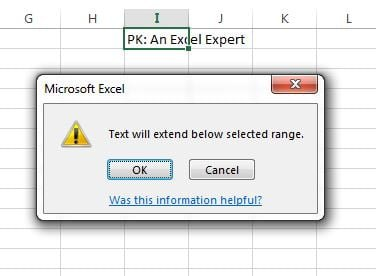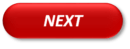# Chapter-1: Fill-Down,Up,Left,Right,Justify and Series

In this lesson you will learn how fill-down, fill-up, fill-left, fill-right, fill justify and fill series. Fill option is available in Home Tab in Editing group.

### Fill Down:

Fill down is used to fill the data from up side to down side. Shortcut Key is Ctrl+D.

In the below image he have a formula on cell “D2”. We can select the range “D2:D13” and press Ctrl+D to fill down the formula.

Formula will be filled down in the range.

### Fill Up:

Fill Up is used to fill the data from down side to up side. Shortcut Key is Alt+E+I+U.

In the below image we have a formula in cell “D13”. We can select the range “D2:D13” and press Alt+E+I+U to fill up the formula.

Formula will be filled up in the range.

### Fill Right:

Fill Right is used to fill the data from left side to right. Shortcut Key is Ctrl+R.

In the below image he have a formula on cell “B4”. We can select the range “B4:H4” and press Ctrl+R to fill right the formula.

Formula will be filled right in the range.

### Fill Left:

Fill Left is used to fill the data from right side to left. Shortcut Key is Alt+E+I+L.

In the below image he have a formula on cell “H4”. We can select the range “B4:H4” and press Alt+E+I+L to fill left the formula.

Formula will be filled left in the range.

### Fill Series:

Fill series is used to fill the data of a sequence . Shortcut Key is Alt+E+I+S.

For example we want to fill the serial number.

To fill the series below are the steps

• Select the first cell in the range that you want to fill.
• Type the starting value for the series.
• Press Alt+E+I+S

Below given window will be displayed

Under Type, following options are available:

• LinearCreates a series that is calculated by adding the value in the Step value box to each cell value in turn.
• Growth: Creates a series that is calculated by multiplying the value in the Step value box by each cell value in turn.
• Date: Creates a series that fills date values incrementally by the value in the Step value box and is dependent on the unit specified under Date unit.
• AutoFill: Creates a series that produces the same results as dragging the fill handle.

Click on linear, which is default selected in this case.

Click on OK button to fill the serial numbers.

### Fill Justify:

Fill Justify is used to fill the text in below cells if it is not fit in the cells . Shortcut Key is Alt+E+I+J.

For example we have a text PK: An Excel Expert in cell “I2” and It is not fill the cell.

Select the Cell “I2” and press Alt+E+I+J